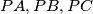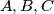### IMO Shortlist 1978 problem 13

Kvaliteta:
Avg: 0,0
Težina:
Avg: 0,0
We consider a fixed point$P$ in the interior of a fixed sphere$.$ We construct three segments$PA, PB,PC$, perpendicular two by two$,$ with the vertexes$A, B, C$ on the sphere$.$ We consider the vertex$Q$ which is opposite to$P$ in the parallelepiped (with right angles) with$PA, PB, PC$ as edges$.$ Find the locus of the point$Q$ when$A, B, C$ take all the positions compatible with our problem.
Izvor: Međunarodna matematička olimpijada, shortlist 1978